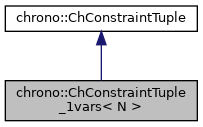chrono::ChConstraintTuple_1vars< T > Class Template Reference

## Description

### template<class T> class chrono::ChConstraintTuple_1vars< T >

This is a container for 'half' of a constraint, and contains a tuple of 1 or 2 or 3 differently-sized jacobian chunks.

For instance, this might happen because you want a constraint between an edge (i.e. two xyz variables, each per end nodes) and a triangle face (i.e. three xyz variables, each per corner), so the jacobian row matrix is split in 2 + 3 chunks, here as two tuples. The complete constraint, ChConstraintTwoTuples, will use two of these classes. Template T is a class of ChVariableTupleCarrier_Nvars type

#include <ChConstraintTuple.h>

Collaboration diagram for chrono::ChConstraintTuple_1vars< T >:[legend]

## Public Member Functions

ChConstraintTuple_1vars ()
Default constructor.

ChConstraintTuple_1vars (const ChConstraintTuple_1vars &other)
Copy constructor.

ChConstraintTuple_1varsoperator= (const ChConstraintTuple_1vars &other)
Assignment operator: copy from other object.

ChRowVectorRef Get_Cq ()

ChVectorRef Get_Eq ()

ChVariablesGetVariables ()

void SetVariables (T &m_tuple_carrier)

void Update_auxiliary (double &g_i)

double Compute_Cq_q ()

void Increment_q (const double deltal)

void MultiplyAndAdd (double &result, const ChVectorDynamic< double > &vect) const

void MultiplyTandAdd (ChVectorDynamic< double > &result, double l)

void Build_Cq (ChSparseMatrix &storage, int insrow)

void Build_CqT (ChSparseMatrix &storage, int inscol)

## Protected Attributes

ChVariablesvariables
The constrained object.

ChRowVectorN< double, T::nvars1 > Cq
The [Cq] jacobian of the constraint.

ChVectorN< double, T::nvars1 > Eq
The [Eq] product [Eq]=[invM]*[Cq]'.

The documentation for this class was generated from the following file:
• /builds/uwsbel/chrono/src/chrono/solver/ChConstraintTuple.h